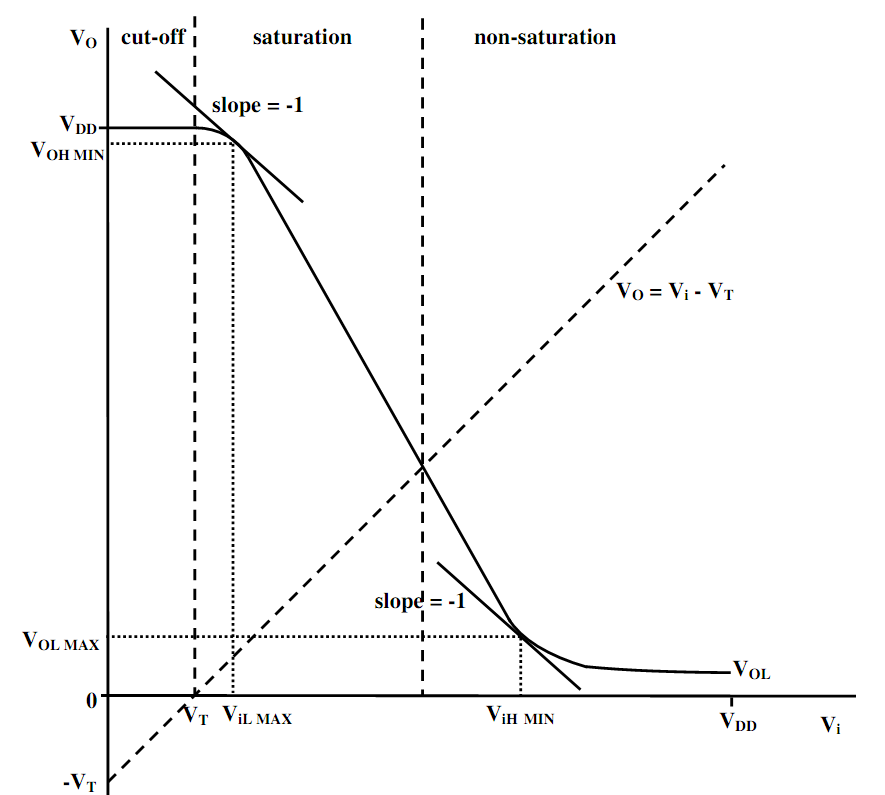Calculate the output voltage of MOS transistor

The transfer characteristic of a particular resistively loaded MOS transistor inverter is demonstrated below in figure. Express an expression for the co-ordinates of the point, (ViA,VOA) upon this characteristic whether VO = Vi. This can be assumed as KnRD >>1 and the consequences of channel length modulation can be avoided.

Calculated the value of VO at particular point when Kn = 100µAV-1, RD = 100kΩ and VT = 1V as well as VDD = 10V.#### Related Questions in Electrical Engineering

• ##### Q :Types of Frequency Modulation Explain

Explain types of the Frequency Modulation?

• ##### Q :Use of Multiple Semaphores Explain

Explain about the use of Multiple Semaphores.

• ##### Q :Schedulability and its types What are

What are schedulability and its types?

• ##### Q :Error correcting methods Specify the

Specify the types of error correcting methods.

• ##### Q :What is invertible system Explain the

Explain the term invertible system?

• ##### Q :Find out the impedance Find out the

Find out the impedance of the network demonstrated below as considered by the voltage source, V1, while operating at a frequency of 1 kHz. Express it in the form of phase and magnitude. Moreover, find the current drawn by the source by the network in simila

• ##### Q :Determine inductor value to accurate

Find out the Power Factor of the alternating current network demonstrated below, while operating at a frequency of 50Hz. Thus, establish a revised value for the inductor, L that will accurate the Power Factor of the network to unity.

##### Q :NAND and NOR gate Explain why NAND gate

Explain why NAND gate is preferred over the NOR gate for fabrication?

• ##### Q :Illustrates the list of the states of

Illustrates the list of the states of timer?

• ##### Q :Explain Embedded System Normal 0 false

Normal 0 false false